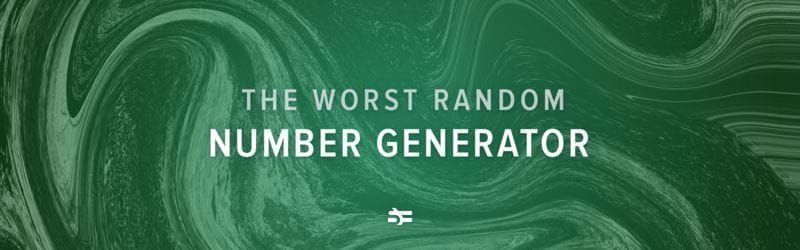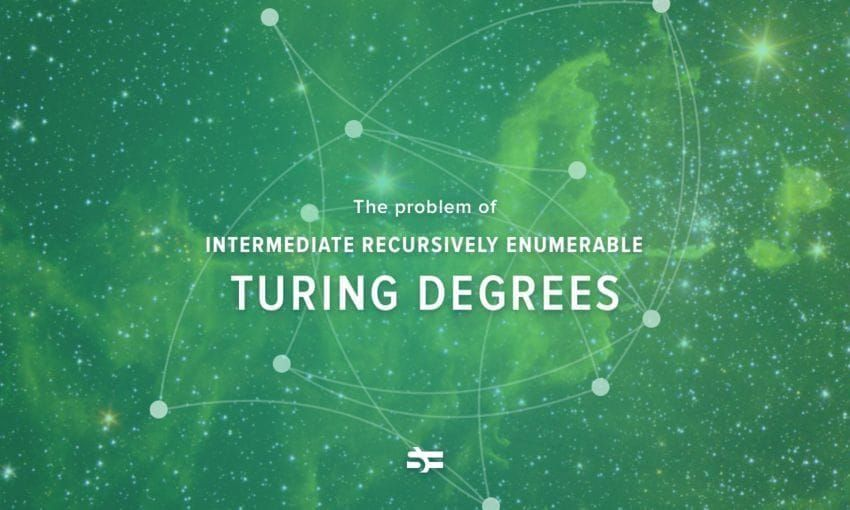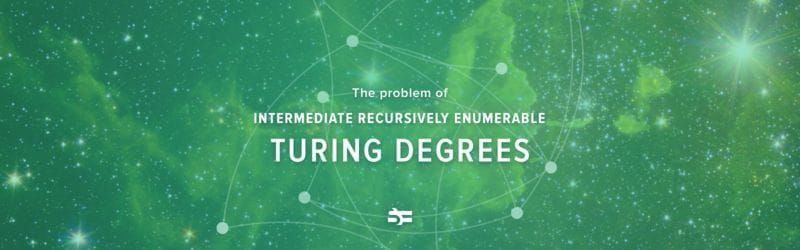# Insane Properties of the Closed Long Ray and the Long Line

###### In topology, the long line, or Alexandroff line, is a space somewhat similar to the real line, but ‘longer’. To obtain the long line, one needs to put together a long ray in each direction. Closed long rays, as well as long lines, have remarkable properties. And this is the subject of a new blog post written by our friends from the ‘Mind vs Trash’ VK-community.

Let us take an interval $[0,1)$ of real numbers and lengthen it with the same interval to the right; now we have $[0,2)$. Nonetheless, nothing has changed topologically: $f : [0, 1) \to [0, 2)$, such that $f(x) = 2 x$, is a homeomorphism. Adding one more such an interval will result in [0,3) which is still homeomorphic, i.e., topologically equivalent to $[0,1)$.

Now, let us combine an infinite number of such intervals. For every natural number, let us take a copy of $[0,1)$ and concatenate them. More precisely: let us take a Cartesian product $\mathbb{N} \times [0,1)$ and with the lexicographic order – for $(n,x)$ pairs we first compare $n$ and then $x$. If we interpret elements of a pair as integral and fractional components of a real number, we can say that we have built an infinite ray $[0, \infty)$. Sadly, we still have not got anything new, as rays $[0,\infty)$ and $[0,1)$ are homeomorphic, for example, by mapping $x \mapsto \frac{x}{x + 1}$.

Let us not despair and add more and more intervals until we obtain something that differs from [0,1). But we will have to keep adding it infinitely, or uncountably. It can be shown that for any countable ordinal $\alpha$ a set $\alpha \times [0, 1)$ with the lexicographic order is homeomorphic to $[0,1)$.

But there are uncountable ordinals as well. Let us take the smallest uncountable ordinal expressed as $\omega_1$ and build $\omega_1 \times [0, 1)$ the same way. The obtained space is called the closed long ray and serves as one of the basic counterexamples of topology. Here are several of its remarkable properties:

• Every increasing sequence in the closed ong ray is convergent.
• The space is sequentially compact (every sequence of points in it has a convergent subsequence converging to a point in it), but it is not compact.
• It is not even a Lindelöf space: open covers do not have a countable subcover (there is no finite subcover as well, otherwise it would imply compactness from the previous paragraph).
• The space is not metrisable, i.e., we cannot define the topology by the metric, as we are used to when dealing with real numbers.
• The space is not separable, unlike real numbers where rational numbers appear as a countable everywhere dense set.
• It is connected but not path-connected because the long line is ‘too long’ to be covered by a path, which is a continuous image of an interval.

By removing the smallest element (0,0) from the closed long ray, we obtain the open long ray. By attaching the reversed open long ray to the left of it, we obtain the long line. The aforesaid spaces have the same insane properties as the closed long ray.

References

Do you like the subject? Then read more articles from the mathematical series in our blog.More from Serokell